# 基于含权Newman算法的交通控制子区划分Traffic Control Subarea Partition Based on Weighted Newman Algorithms

DOI: 10.12677/OJTT.2019.82018, PDF, HTML, XML, 下载: 592  浏览: 1,692

Abstract: A dual network is structured firstly by setting link of road network as dual node and incidence relationship between links of road network as dual links in the dual network in order to keep homogeneity of traffic flow in each road sub-network partitioned. Link traffic flow, which is the result of traffic demand assignment based on user equilibrium principle, is divided by link length and then the definition of quasi traffic density of each link is proposed secondly. Newman network partition algorithm is extended by introducing the definition of quasi traffic density. Thirdly, the superiority of K-means cluster algorithm, Newman network partition algorithm and extended Newman network partition algorithm are identified by measurement of Normalized cut Silhouette (NSK) on a real road network. This study has identified that: indexes value NS and NSK  on networks of traditional K-means cluster algorithm are relatively small, but the sub-network partitioned is not connected in the space so that it lacks practical value. Index  NSK of extended Newman network partition algorithm is prior to that of Newman network partition algorithm. The sub-network which is partitioned by extended Newman network partition algorithm introduced the definition of quasi traffic density is favorable for purpose of traffic flow control.

1. 引言

2. Newman快速划分算法及其改进

$Q=\underset{ij}{\sum }\left[\frac{{A}_{ij}}{2m}-\frac{{k}_{i}*{k}_{j}}{\left(2m\right)\left(2m\right)}\right]{\delta }_{\left({C}_{i},{C}_{i}\right)}$ (1)

$Q=\underset{i}{\sum }\left({e}_{ii}-{a}_{i}{}^{2}\right)=\text{Tre}-‖{e}^{2}‖$ (2)

${e}^{2}=\underset{i}{\sum }{a}_{i}^{2}=\underset{i}{\sum }{\sum }_{j,k}{e}_{ij}{e}_{ik}$ (3)

2.1. 基于模块度的Newman快速划分算法

2.2. 算法步骤

Newman快速算法执行过程如下：

1) 首先把含有 $n$ 个的节点复杂网络视为 $n$ 个社团，那么，初始时有：

 (4)

${a}_{i}=\frac{{k}_{i}}{2m}$ (5)

2) 根据贪婪算法的原理，沿着使 $Q$ 增加或者减小速度最小的方向，依次合并两个有边连接的社团，并计算合并后的 $Q$ 值增量：

$\Delta Q={e}_{ij}+{e}_{ji}-2{a}_{i}{a}_{j}=2\left({e}_{ij}-{a}_{i}{a}_{j}\right)$ (6)

3) 重复上一步，直到每个节点都合并成为一个社团为止。

2.3. 算法改进

${D}_{ij}=1-\frac{|{d}_{i}-{d}_{j}|}{{\sum }^{\text{​}}{d}_{i}}$ (7)

${e}_{ij}=\left\{\begin{array}{l}\frac{{D}_{ij}}{{\sum }_{i,j}{D}_{ij}};节点i与节点j之间有边相连\\ 0;其他\end{array}$ (8)

${a}_{i}$ 可以表示为：

${a}_{i}=\frac{{\sum }_{j}{e}_{ij}}{{\sum }_{i,j}{D}_{ij}}$ (9)

3. 实例分析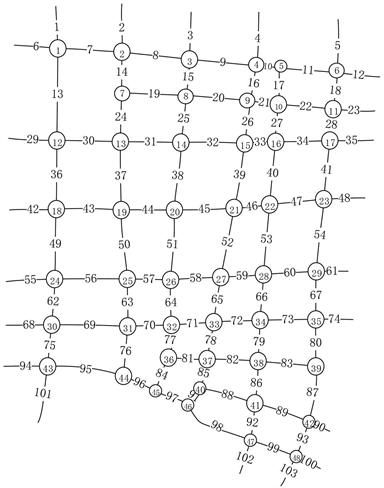Figure 1. Changde downtown urban road network map

3.1. 子区划分结果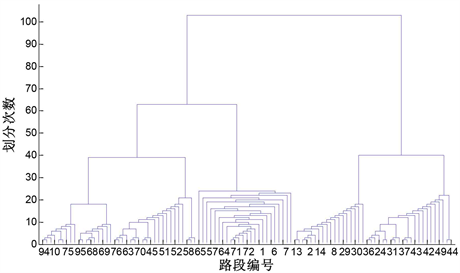Figure 2. Controls sub-area partitioning tree diagram based on Traditional Newman algorithm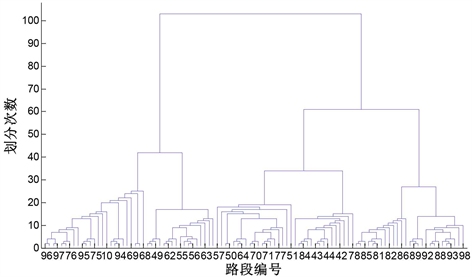Figure 3. Controls sub-area partitioning tree diagram based on Improved Newman algorithm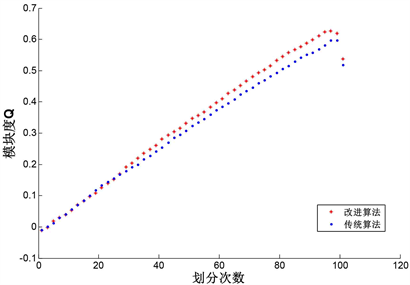Figure 4. Modular values of subdivision results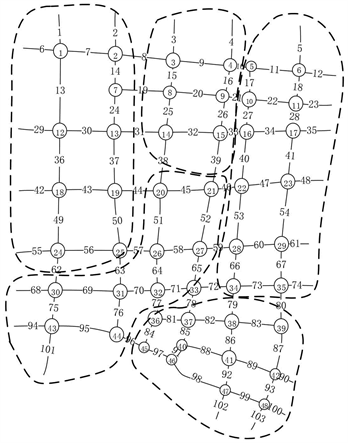Figure 5. Control sub-area division result based on Traditional Newman algorithm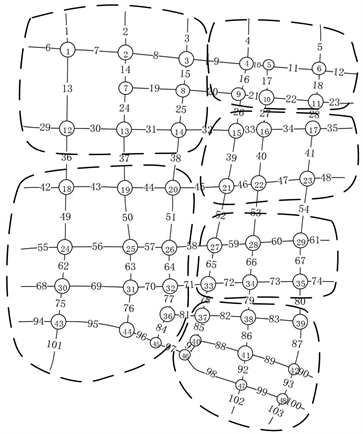Figure 6. Control sub-area division result based on Improved Newman algorithm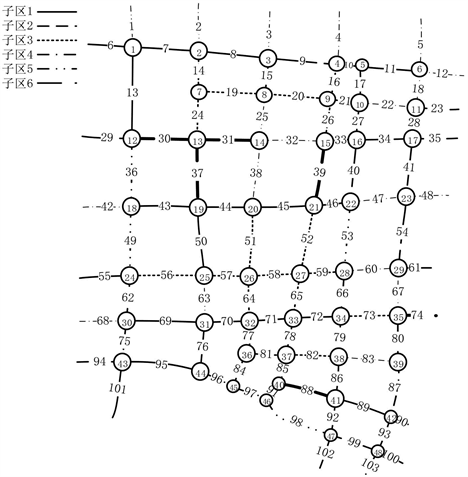Figure 7. First sub-division based on K-means clustering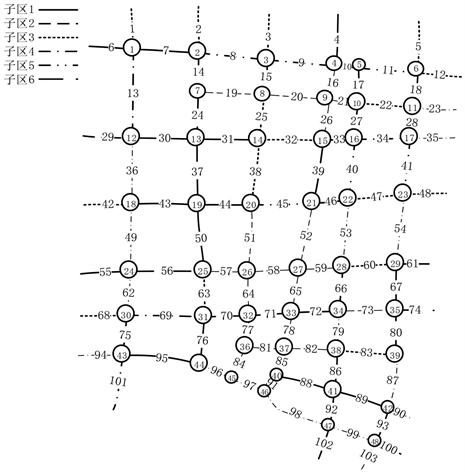Figure 8. Second sub-division based on K-means clustering

3.2. 划分结果指标分析

$N{S}_{k}\left(A,B\right)=\frac{{\sum }_{i\in A}{\sum }_{j\in B}{\left({d}_{i}-{d}_{j}\right)}^{2}}{{N}_{A}{N}_{B}}$ (10)

${d}_{i}$ 表示路段 $i$ 的密度， ${N}_{A},{N}_{B}$ 分别为子区域A和子区域B路段的数目。由于 $N{S}_{k}\left(A,B\right)$ 仅表征了子区域A和子区域B之间的平均密度距离，并不能体现子区之间的空间关系。为了补充这一点，进而采用公式(11)对其进行评价：

$N{S}_{k}\left(A\right)=\frac{N{S}_{k}\left(A,A\right)}{N{S}_{k}\left(A,B\right)}$ (11)

$N{S}_{k}\left(A,B\right)=\mathrm{min}\left\{N{S}_{k}\left(A,K\right)|K\in Neighbor\left(A\right)\right\}$ (12)

$AN{S}_{k}=\frac{{\sum }_{A\in C}N{S}_{k}\left(A\right)}{k}$ (13)

$NS$ 评价标准也可以以路段拟交通流密度的方差和均值来表达：

$N{S}_{k}\left(A,B\right)=Var\left(A\right)+Var\left(B\right)+{\left({u}_{A}-{u}_{B}\right)}^{2}$ (14)

$N{S}_{k}\left(A\right)=\frac{N{S}_{k}\left(A,A\right)}{N{S}_{k}\left(A,B\right)}=\frac{2Var\left(A\right)}{Var\left(A\right)+Var\left(B\right)+{\left({u}_{A}-{u}_{B}\right)}^{2}}$ (15)Table 1. Evaluation index of various zoning methods

4. 小结

  Walinchus, R.J. (1971) Real-Time Network Decomposition and Sub Network Interfacing. Highway Research Record, 7, 20-28.  Ji, Y. and Geroliminis, N. (2012) On the Spatial Partitioning of Urban Transportation Networks. Transportation Research Part B: Methodological, 16, 1639-1656. https://doi.org/10.1016/j.trb.2012.08.005  Ji, Y. and Geroliminis, N. (2011) Spatial and Temporal Analysis of Congestion in Urban Transportation Networks. Trans-portation Research Board Annual Meeting, 1791-1808.  李刚奇, 赵娅丽. 基于宏观交通理论的交通控制子区划分方法[C]//第七届中国智能交通年会. 第七届中国智能交通年会优秀论文集——智能交通技术. 北京: 中国智能交通协会, 2012: 31-38.  马莹莹, 杨晓光, 曾澄. 基于谱方法的城市交通信号控制网络小区划分方法[J]. 系统工程理论与实践, 2010, 30(12): 2290-2296.  卢守峰, 陶黎明, 江勇东. 考虑连接性的路网划分算法[J]. 交通运输系统工程与信息, 2018, 18(5): 95-102.  Dimitriou, L. and Nikolaou, P. (2017) Dynamic Partitioning of Urban Road Networks Haled on Their Topological Andoperational Characteristics. IEEE International Conference on MODELS and Technologies for Intelligent Transportation Systems, Naples, Italy, 26-28 June 2017, 457-462.  王晓轩. 基于聚类的城市交通路网分区和交通状态判别[D]: [硕士学位论文]. 北京: 北京交通大学, 2017.  Newman, M.E.J. and Girvan, M. (2004) Finding and Evaluating Community Structure in Networks. Physical Review E, 69, Article ID: 026113. https://doi.org/10.1103/PhysRevE.69.026113  Girvan, M. and Newman, M.E.J. (2002) Community Structure in Social and Biological Networks. Proceedings of the National Academy of Sciences of the United States of America, 99, 7821-7826. https://doi.org/10.1073/pnas.122653799  Ji, Y.X. and Geroliminis N. (2012) On the Spatial Partitioning of Urban Transportation Networks. Transportation Research Part B, 46, 1639-1656. https://doi.org/10.1016/j.trb.2012.08.005  Kernighan, B.W. and Lin, S. (1970) An Efficient Heuristic Procedure for Partitioning Graphs. The Bell System Technical Journal, 49, 291-307. https://doi.org/10.1002/j.1538-7305.1970.tb01770.x  Newman, M.E.J. (2004) Detecting Community Structure in Networks. European Physical Journal B, 38, 321-330. https://doi.org/10.1140/epjb/e2004-00124-y  刘澜, 卢维科, 胡国静, 等. 面向边界控制地路网小区划分[J]. 中国公路学报, 2018, 31(11): 187-196.